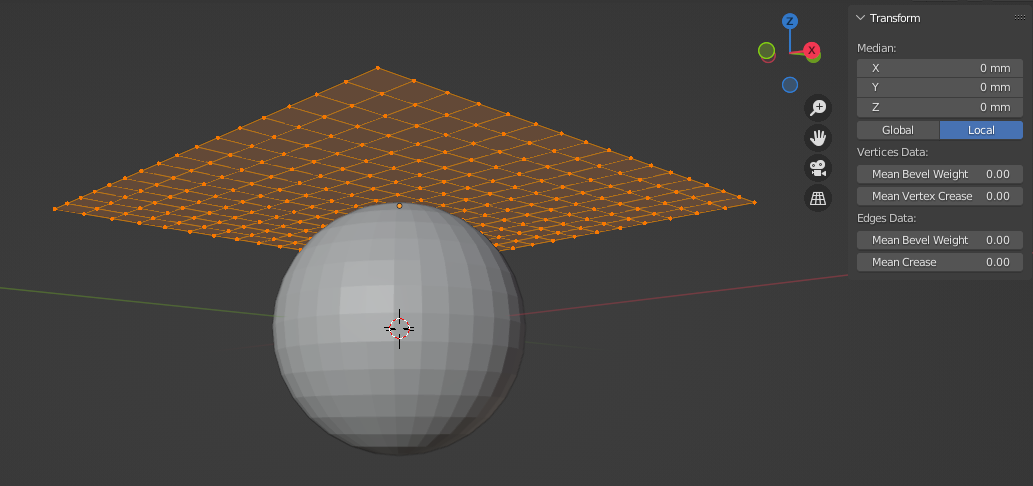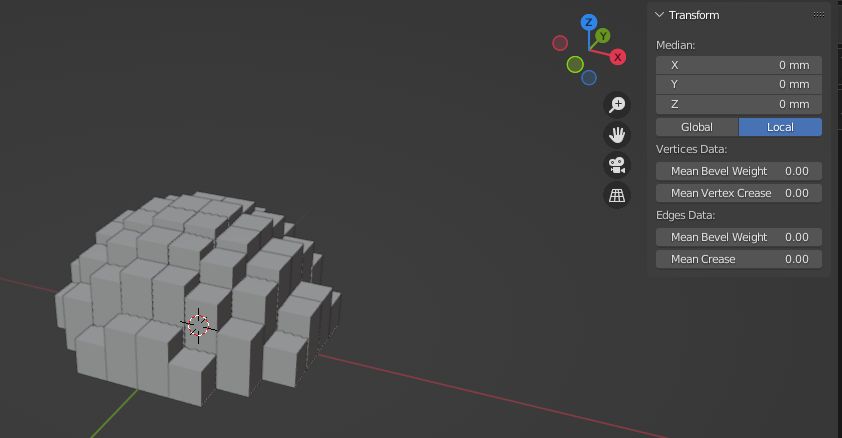# Ray Cast from specific fixed faces of a grid to a moved object giving the wrong grid face IDs

I am trying to get the world point coordinates of a moved object’s raycast (scale, rotation and translation). I have created a grid object and each face location correlates to the raycast’s origin. My code iterated through the grid faces and uses the face center points as the input for the ray_cast function as seen below.

``````        for f in bpy.data.objects['Grid'].data.polygons:
result, location, normal, index = obj_depsgraph.ray_cast(
origin = f.center,
direction = [0,0,1],
distance = bpy.data.objects['Grid'].location.z,
depsgraph = depsgraph)
voxel_XYZ_coordinates [f.index] = location
if result == True:
size = self.voxel_size,
align = 'WORLD',
location = (location,location,location/2),  # Must optimize this formula
scale = (1,1,1),
)
bpy.context.active_object.dimensions.z = location
i += 1
print ("HITs",i, "at face", f.index,"in", f.center)
``````

The setup initially looks like thisand once it runs the result looks like thisAs you can see I am doing a voxelization script that only takes the top surface heights and creates 1 voxel per location in the grid.

So good news is that the code works if I don’t move the object AND (this is the important bit) the face IDs, which have a specific X and Y component, have the correct height assigned to them.

What I don’t want to do, is move those voxels to match the sphere. I want to create voxels in the positions where the grid faces cast a ray downward and hit the sphere. This means that even if the sphere is not perfectly aligned to fit the grid, the voxels are created only in the correct position. Also, again, the face ids from the grid should gather the point values only if they hit the object.

Another way of explaining it would be:

Say I have a grid of 3 x 3 represented as a matrix with zeroes:

``````[0 0 0     ID matrix: [6 7 8
0 0 0                 3 4 5
0 0 0]                1 2 3]
``````

and an object directly below it represented as 1 at 2m(world coordinates):

``````[0 0 0
0 1 0
0 0 0]
``````

In this scenario running the code gives me a HIT in the face ID 4 at (0,0,2) which is correct. Now when I move the object the new height is 3m:

``````[0 0 0
0 0 0
1 0 0]
``````

The code currently gives me. Hit in face ID 4 at (0,0,2). What I would like is: Hit at face ID 1 at (X,Y,3). Where X and Y are the face ID 1 X and Y components.

Hope this all makes sense.

Thanks a lot!

D